# Difference between revisions of "Virial pressure"

The virial pressure is commonly used to obtain the pressure from a general simulation. It is particularly well suited to molecular dynamics, since forces are evaluated and readily available. For pair interactions, one has: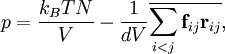$p = \frac{ k_B T N}{V} - \frac{ 1 }{ d V } \overline{ \sum_{i

where one can recognize an ideal term, and a second term due to the virial. The overline is an average, which would be a time average in molecular dynamics, or an ensemble average in Monte Carlo;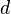$d$ is the dimension of the system (3 in the "real" world).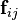${\mathbf f}_{ij}$ is the force on particle$i$ exerted by particle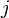$j$, and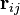${\mathbf r}_{ij}$ is the vector going from$i$ to$j$: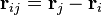${\mathbf r}_{ij} = {\mathbf r}_j - {\mathbf r}_i$.

This relationship is readily obtained by writing the partition function in "reduced coordinates"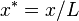$x^*=x/L$, etc, then considering a "blow-up" of the system by changing the value of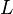$L$. This would apply to a simple cubic system, but the same ideas can also be applied to obtain expressions for the stress tensor and the surface tension, and are also used in constant-pressure Monte Carlo.

If the interaction is central, the force is given by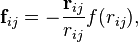${\mathbf f}_{ij} = - \frac{{\mathbf r}_{ij}}{ r_{ij}} f(r_{ij}) ,$

where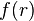$f(r)$ the force corresponding to the intermolecular potential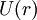$U(r)$: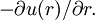$-\partial u(r)/\partial r.$

E.g., for the Lennard-Jones potential,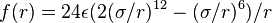$f(r)=24\epsilon(2(\sigma/r)^{12}- (\sigma/r)^6 )/r$. Hence, the expression reduces to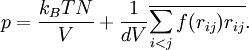$p = \frac{ k_B T N}{V} + \frac{ 1 }{ d V } \overline{ \sum_{i